Kinematic Self-Replicating Machines

Robert A. Freitas Jr., Ralph C. Merkle, Kinematic Self-Replicating Machines, Landes Bioscience, Georgetown, TX, 2004.

5.9.1 Fibonacci's Rabbits

Leonardo Fibonacci (1175-1250) was the greatest European mathematician of the Middle Ages. His full name was Leonardo of Pisa, or Leonardo Pisano in Italian since he was born in Pisa, the city with the famous Leaning Tower . In his first book , originally published in 1202, Fibonacci introduces the following problem:

A pair of rabbits are put in a field and, if rabbits take a month to become mature and then produce a new pair every month after that, how many pairs will there be in twelve months time?

In his solution to this puzzle, Fibonacci assumes that no rabbits ever escape and none die, and no extra rabbits are introduced into the field except those born from this original pair. He puts a newborn pair of rabbits, one male, one female, in a field and allows the rabbits to mate at the age of one month so that at the end of its second month a female can produce another pair of rabbits. He further assumes that the female always produces one new pair (one male, one female) every month from the second month on. The course of rabbit reproduction, illustrated in Figure 5.9, proceeds as follows :

Start of Month 1: The rabbits are placed in the field but have not mated; there is only 1 pair.
End of Month 1: The rabbits mate; there is only 1 pair in the field.
End of Month 2: The female produces one new pair; there are now 2 pairs in the field.
End of Month 3: The original female produces a second pair; there are now 3 pairs in the field.
End of Month 4: The original female has produced yet another new pair, the female born two months ago produces her first pair also; there are now 5 pairs in the field.
End of Month 5: ...and so on, adding the latest two numbers to get the next one.

The total number of pairs of rabbits in the field at the start of each month, counting from month n = 1, is: 1, 1, 2, 3, 5, 8, 13, 21, 34, 55, 89, 144, and there are 233 pairs at the end of month 12. The French mathematician Edouard Lucas (1842-1891) [2830-2832] first gave the name “Fibonacci numbers” to this series. The Fibonacci sequence appears extensively in nature [2833, 2834], especially in botany.

To express this progression mathematically as a recurrence relation , we define F(n) as the number of pairs of rabbits in the field at the start of month n. Then F(0) = 0, F(1) = 1, and: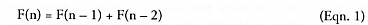for n > 1. The number of pairs at the end of month n is simply: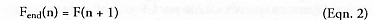and the total elapsed time is t = nt, where t is the replication (or gestation) time, the units of time required for each replication cycle to be completed. t = 1 month in this example.

Interestingly, the ratio of successive Fibonacci numbers, F(n – 1) / F(n – 2), approaches a constant value f as n → ∞. The constant f (phi) is often called the golden mean (Euclid ), the golden section (Leonardo da Vinci), the golden ratio, or the golden number (see value, below). f is given explicitly by the relation: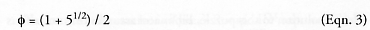and according to Binet’s formula for the nth Fibonacci number (apparently actually devised first by the mathematician A. de Moivre in 1730, more than a century before J.P.M. Binet rediscovered it in 1843 ), the nth value of the Fibonacci series is given exactly by: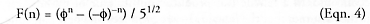for all positive integers n, where f = 1.61803 39887 49894 84820 45868 34365 63811 77203 09179 80576 .... Knott  points out that since abs(– (–f)–n) < 1 for n > 0, the influence of this term decreases rapidly with increasing n, allowing the exact formula to be simplified further to: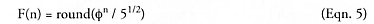where the “round” function gives the nearest integer to its argument.

The rabbit formula (Eqn. 4) assumes that newborn rabbits are “birth-immature replicators” – they require some length of time (one cycle in this example) to mature before they can begin reproducing at the rate of one offspring per cycle. Taneja and Walsh  have generalized the rabbit formula to allow for a variable maturation time tmat, where tmat/t = 1, 2, 3, 4, ..., showing that the number of pairs at the end of n cycles is: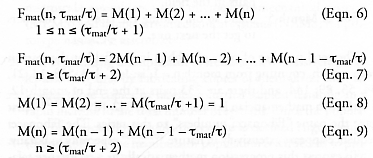where M(n) is the number of adult replicators (capable of replication) in the nth cycle. Birth-immature replicators that require more than one cycle to reach maturity may be called “extended-maturation replicators.” Note that human beings are very extended maturation replicators, with tmat/t ~ 17.0 currently in the United States, assuming a replicator gestation time of t = 0.736 year (~9 months) and a replicator maturation time of tmat = 12.54 years, the average age of menarche (first menstruation) for U.S. females during 1988-1994  (cf. 12.75 years during 1963-1970 in U.S. , 12.9 years in U.K. , and from 12-18 years across various cultures and throughout history ).

Phares and Simmons  have derived a sum rule for the generalized form of the Eqn. 1 recurrence relation F(n) = a·F(n – 1) + b·F(n – 2), where a and b are arbitrary constant coefficients that could perhaps be used to represent post-pregnancy alterations in replicator fecundity.

Last updated on 1 August 2005